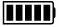ECE4253 Digital Communications Department of Electrical and Computer Engineering - University of New Brunswick, Fredericton, NB, Canada

# Galois Field GF(2) Calculator

 Model C-172 POLYNOMIAL CALCULATORA:

B:

 A + B A - B A × B A / B

Discussion  Polynomials  MATLAB

``` 110010100100000111
-------------------- = 110110010101, Remainder = 000100
1001111```

## Polynomial Form

 ``` x17+x16+x13+x11+x8+x2+x+1 ----------------------- = x11+x10+x8+x7+x4+x2+1, Remainder: x2 x6+x3+x2+x+1```

# MATLAB Example

Calculations in GF(2) are performed by MATLAB functions found within the Communications Toolbox.

>> a = gf( [1 1 0 0 1 0 1 0 0 1 0 0 0 0 0 1 1 1] );

>> b = gf( [1 0 0 1 1 1 1] );

>> [q,r] = deconv(a,b) % deconvolution is equivalent to division

q = GF(2) array.

Array elements =

1      1      0      1      1      0      0      1      0      1      0      1

r = GF(2) array.

Array elements =

0      0      0      0      1      0      0

 2021-10-25 00:38:35 ADT Last Updated: 2010-04-29 Richard Tervo [ tervo@unb.ca ]